# pointCloud

Object for storing 3-D point cloud

## Description

The `pointCloud` object creates point cloud data from a set of points in 3-D coordinate system. The points generally represent the x,y, and z geometric coordinates for samples on a surface or of an environment. Each point can also be represented with additional information, such as the RGB color. The point cloud data is stored as an object with the properties listed in Properties. Use Object Functions to retrieve, select, and remove desired points from the point cloud data.

## Creation

### Syntax

``ptCloud = pointCloud(xyzPoints)``
``ptCloud = pointCloud(___,Name=Value)``

### Description

````ptCloud = pointCloud(xyzPoints)` returns a point cloud object with coordinates specified by `xyzPoints`.```

example

````ptCloud = pointCloud(___,Name=Value)` sets options using one or more name-value arguments in addition to the previous syntax. For example, `Color=[1 0 0]` sets the color of the point cloud to red.```

### Input Arguments

expand all

3-D coordinate points, specified as an M-by-3 list of points or an M-by-N-by-3 array for an organized point cloud. The 3-D coordinate points specify the x, y, and z positions of a point in the 3-D coordinate space. The first two dimensions of an organized point cloud correspond to the scanning order from sensors such as RGBD or lidar. This argument sets the `Location` property.

Data Types: `single` | `double`

Name-Value Arguments

Specify optional pairs of arguments as `Name1=Value1,...,NameN=ValueN`, where `Name` is the argument name and `Value` is the corresponding value. Name-value arguments must appear after other arguments, but the order of the pairs does not matter.

Before R2021a, use commas to separate each name and value, and enclose `Name` in quotes.

Example: `Color=[1 0 0]` sets the color of the point cloud to red.

Point cloud color, specified as an RGB value as one of, a color string, a 1-by-3 vector, or an M-by-3 or M-by-N-by-3 matrix. M-by- N specifies the dimensions of the point cloud. Each entry specifies the RGB color of a point in the point cloud data. Therefore, you can specify the same color for all points or a different color for each point.

• For `single` or `double` datatype, the `Color` RGB value must be specified in the range [0, 1].

• For `uint8` datatype, the `Color` RGB value must be specified in the range [0, 255].

• For `uint16` datatype, the `Color` RGB value must be specified in the range [0, 65535].

• The function stores the `Color` value as either an M-by-3 or M-by-N-by-3. If you specify the color as a string or a 1-by-3 vector, the function converts it to one of these dimensions.

ColorFormatExample
Specify one color for all points

string scalar or character vector

`"r"`

`"red"`

1-by-3 vector

`[255 0 0]`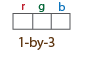Specify a color for each point

cell array of character vectors

`["red","yellow","blue"]`

M-by-3 matrix, as a list of RGB values

```255 0 0 255 0 0 0 255 255```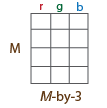M-by-N-by-3 matrix for an organized point cloud containing RGB values for each point, where M-by-N is the dimension of the point cloud.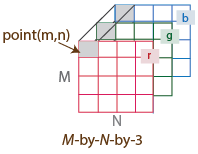Data Types: `uint8` | `uint16`

Surface normals, specified as a M-by-3 or M-by-N-by-3 array. Use this property to specify the normal vector with respect to each point in the point cloud. Each entry in the surface normals specifies the x, y, and z component of a normal vector.

CoordinatesSurface Normals
M-by-3 arrayM-by-3 array, where each row contains a corresponding normal vector.
M-by-N-by-3 arrayM-by-N-by-3 array containing a 1-by-1-by-3 normal vector for each point.

Data Types: `single` | `double`

Grayscale intensities at each point, specified as a M-by-1 vector or M-by-N matrix. The function maps each intensity value to a color value in the current colormap.

CoordinatesIntensity
M-by-3 arrayM-by-1 vector, where each row contains a corresponding intensity value.
M-by-N-by-3 arrayM-by-N matrix containing intensity value for each point.

Data Types: `single` | `double` | `uint8` | `uint16`

### Output Arguments

expand all

Point cloud, returned as a `pointCloud` object with the properties listed in Properties.

## Properties

expand all

Position of the points in 3-D coordinate space, specified as an M-by-3 or M-by-N-by-3 array. Each entry specifies the x, y, and z coordinates of a point in the 3-D coordinate space. The `xyzPoints` input argument sets this property. To reduce memory use, you can store point locations as a `single` data type.

• For unorganized point clouds, `Location` must be specified as an M-by-3 array, where M is the total number of points, and the array provides the x,y,z coordinates for each point.

• For organized point clouds, `Location` must be specified as an M-by-N-by-3 array, where M*N is the total number of points, and the array provides the x,y,z coordinates for each point. Points obtained from a projective camera, such as Kinect® or a lidar sensor, are stored as an organized point cloud. An organized point cloud is laid out as a 2-D array of points that resemble an image-like structure.

Data Types: `single` | `double`

Number of points in the point cloud, stored as a positive integer.

Range of coordinates along x-axis, stored as a 1-by-2 vector.

Range of coordinates along y-axis, stored as a 1-by-2 vector.

Range of coordinates along z-axis, stored as a 1-by-2 vector.

## Object Functions

 `findNearestNeighbors` Find nearest neighbors of a point in point cloud `findNeighborsInRadius` Find neighbors within a radius of a point in the point cloud `findPointsInROI` Find points within a region of interest in the point cloud `findPointsInCylinder` Find points within a cylindrical region in a point cloud `removeInvalidPoints` Remove invalid points from point cloud `select` Select points in point cloud `copy` Copy array of point cloud objects

## Examples

collapse all

Read the 3-D coordinate points into the workspace.

`load("xyzPoints");`

Create a point cloud object from the input point coordinates.

`ptCloud = pointCloud(xyzPoints);`

Inspect the properties of the point cloud object.

`ptCloud`
```ptCloud = pointCloud with properties: Location: [5184x3 single] Count: 5184 XLimits: [-3 3.4338] YLimits: [-2 2] ZLimits: [0.0016 3.1437] Color: [] Normal: [] Intensity: [] ```

Display the point cloud by using `pcshow`.

`pcshow(ptCloud)`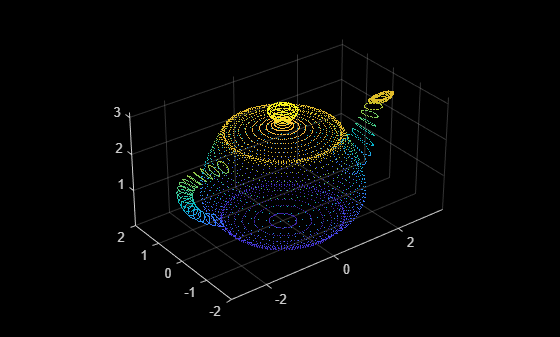Modify Color of Point Cloud Data

Create an RGB color array of size same as the size of the point cloud data. Set the point colors to Red.

`cmatrix = ones(size(ptCloud.Location)).*[1 0 0];`

Create the point cloud object with the color property set to the RGB color array.

```ptCloud = pointCloud(xyzPoints,Color=cmatrix); pcshow(ptCloud)```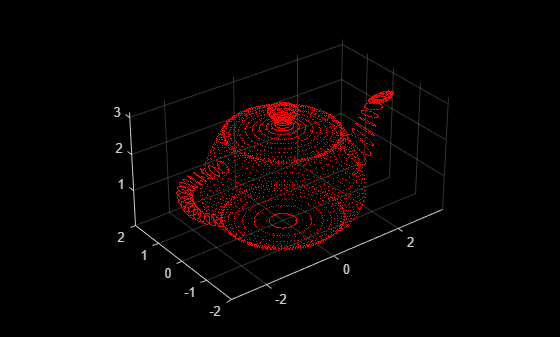Add Surface Normals to Point Cloud Data

Compute surface normals corresponding to the point cloud data using `pcnormals`.

`normals = pcnormals(ptCloud);`

Create point cloud object from input point coordinates. Add the computed surface normals to point cloud object.

`ptCloud = pointCloud(xyzPoints,Normal=normals);`

Display the point cloud and plot the surface normals.

```pcshow(ptCloud) x = ptCloud.Location(:,1); y = ptCloud.Location(:,2); z = ptCloud.Location(:,3); u = normals(:,1); v = normals(:,2); w = normals(:,3); hold on quiver3(x,y,z,u,v,w); hold off```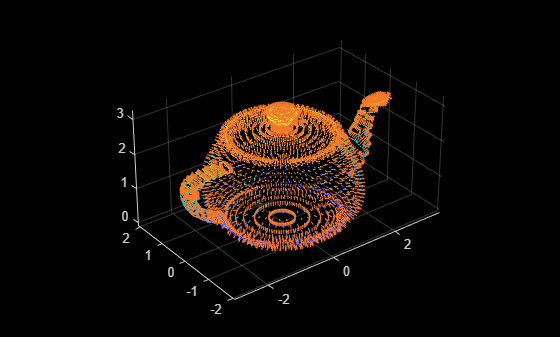## Tips

The `pointCloud` object is a `handle` object. If you want to create a separate copy of a point cloud, you can use the MATLAB® `copy` method.

 `ptCloudB` = `copy`(`ptCloudA`)

If you want to preserve a single copy of a point cloud, which can be modified by point cloud functions, use the same point cloud variable name for the input and output.

 `ptCloud` = pcFunction(`ptCloud`)

## Version History

Introduced in R2015a

expand all